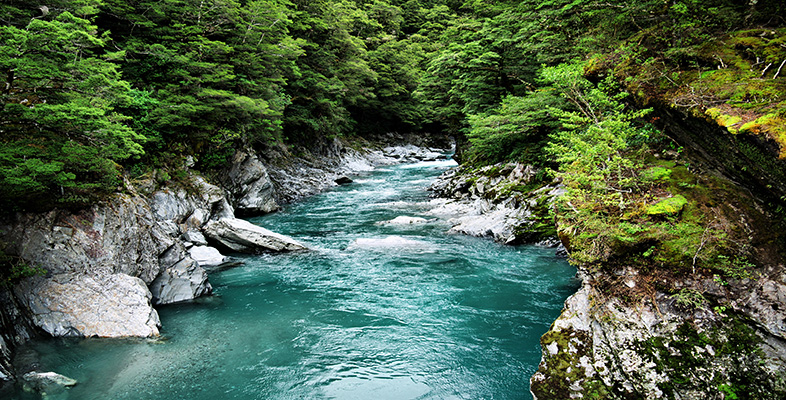Water for life

Start this free course now. Just create an account and sign in. Enrol and complete the course for a free statement of participation or digital badge if available.

Free course

# 2 Earth's store of water

## 2.1 Where water occurs and how we measure it

When astronauts first ventured to the Moon in the late 1960s, they were captivated by a vision of the Earth in colour as it had never been seen before (Figure 2). It is not surprising that, after pictures like this were published, the Earth became known as the 'blue planet'.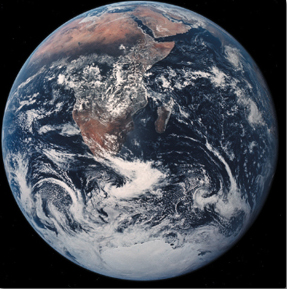Figure 2 A view of the Earth from space. The brown areas are Africa and Arabia, the larger blue areas are the oceans, and the white areas are cloud

It is astonishing how much of the Earth's surface is covered by water. The oceans occupy about 71% of the Earth's surface. Altogether, the total volume of water on Earth, including the oceans, lakes, rivers and what is stored in rocks underground, is estimated to be roughly 1460 000 000 000 000 000 000 (1460 billion billion) litres.

### Question 1

What is the average daily intake of water for a person in the UK (from food as well as drinks)?

Even allowing 5 litres per person to ensure the most basic standard of domestic use (i.e. the minimum for cooking and washing), in addition to what humans take into their bodies, it would be reasonable to imagine that there is enough water around to sustain not only the Earth's 6000 000 000 (6 billion) human inhabitants, but all the other species of animals and plants as well - with an awful lot to spare.

However, there are frequent news bulletins that illustrate the appalling consequences of droughts in some parts of the world. Even countries such as the UK suffer water shortages: for example, in southeast England during the summer of 2006. It seems that the planet is well endowed with life-giving water but, from a human perspective, it is often in the wrong place, in the wrong form (for example, seawater is plentiful in coastal towns but fresh drinking water could be in short supply), or available at the wrong time.

To find out why there are water shortages and droughts on a planet endowed with 1460 billion billion litres of water, it is necessary to look at where the water occurs. First, the numbers need to be made more manageable by introducing a larger unit to measure the volumes. The unit most commonly used for this purpose is the cubic kilometre, abbreviated to km3, which is the volume of a cube with sides 1 km long. One cubic kilometre is equivalent to 1000 000 000 000 litres, or one million million litres, which can be demonstrated as follows.

To discover how many litres (each of which is equivalent to a 10 cm cube) can be stacked up in a 1 km cube: there are 10 lots of 10 centimetres in one metre (since 10 × 10 cm = 100 cm = 1 m), and there are 1000 metres in 1 kilometre, and so there are 10 × 1000 lots, or 10 000 lots, of 10 centimetres in 1 kilometre. This means that 10 000 one-litre cubes could be placed side-by-side along one edge of a one-kilometre cube. So the total number of one litre cubes that could be stacked within a one-kilometre cube is 10 000 × 10 000 × 10 000, which is 1000 000 000 000. The relationship between litres and km3, m3 and cm3 is summarised below.

Units of volume

• 1 km3 = 1000 000 000 000 litres
• 1 m3 = 1000 litres
• 1 cm3 = 1/1000 litre, so
• 1000 cm3 = 1 litre

A cubic kilometre is 1000 000 000 000 times larger than a litre, so the number of cubic kilometres of water on the Earth is 1000 000 000 000 times smaller than the number of litres. This means that the number of cubic kilometres of water on Earth is: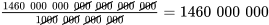Note how 12 zeros have been cancelled out. This makes the number representing the total water volume a little more manageable, and this volume is shown as the bottom line in Table 1, together with the volumes (in km3) stored in each of the Earth's various natural reservoirs. (Don't confuse this use of the term 'reservoir' with the reservoirs that are built to store water for human use.) The volumes listed in Table 1 for ice and snow and for the atmosphere are the volumes of liquid water that would be produced by melting the solid ice and snow and by condensing the water vapour from the atmosphere. It is important to note that all of the volumes shown in Table 1 are estimates; clearly, no one has been able to measure the volume of water in the oceans accurately.

### Table 1 Estimated volumes of water stored in the Earth's natural reservoirs. To be completed as part of Question 1.

ReservoirVolume/km3Volume/% total water
oceans1400 000 00096
ice and snow43 000 000
underground water15 000 0001.0
lakes and rivers360 0000.025
atmosphere15 0000.001
plants and animals2 0000.000 14
total1460 000 000*100*
*The numbers in the middle column add up to 1458 377 000, but they have been rounded to 1460 000 000 to reflect the limited accuracy of the larger numbers. Similarly, when you have filled in the gap in the third column (Question 1), you will find that the numbers do not add up to exactly 100.

Even though the 12 zeros have been removed by expressing the volumes in km3 rather than litres, the numbers in the middle column of Table 1 are still too large to be handled easily. They still have too many zeros. It is much easier to work with percentages of the total water volume in the Earth's natural reservoirs, which are displayed in the right-hand column of the table. You can see that about 96% of the water is stored in the oceans, which means that there is only a small percentage available for human use on the land. To put it another way, if all the Earth's water was represented by the contents of a 4.5 litre (1 gallon) can, all but the contents of a tea cup would be seawater.

### Question 1

There is a gap in the right-hand column of Table 1. Calculate the proportion of water that is stored in ice and snow as a percentage of the total volume of water stored on Earth.

(Hint: to do this, first write down the proportion as a fraction. If the numbers involved are too large to enter on your calculator, you will need to reduce the fraction to an equivalent fraction with smaller numbers on the top and the bottom by cancelling out some zeros. Then use your calculator to work out the required percentage.)

From Table 1, the volume of water stored in ice and snow is 43 000 000 km3. Expressing this as a fraction of the total volume gives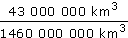Six zeros (and the unit) can be cancelled from the top and the bottom of this fraction, so the percentage is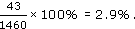(Note that 43 is exactly the same percentage of 1460 as 43 000 000 is of 1460 000 000.)

Percentages are one way to avoid having to work with cumbersome numbers such as those in Table 1, but an alternative is to use a mathematical notation for expressing the numbers in a more convenient form. This notation is called scientific notation and it is based on the observation that every time a number is multiplied by 10, a zero is added to the end of the number. The use of powers of ten and scientific notation is explained in Section 2.2.

S154_1